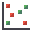RocFall3 | Your new tool for 3D assessment of slopes at risk for rockfalls. Get started with RocFall3 today!

# Scatter Plot

To create a Scatter Plot after a Probabilistic analysis in SWedge:

1. Select Statistics > Plot Scatter or the Plot Scattertoolbar button.
2. Select the Data Type you would like to plot on the X-axis and Y-axis.
3. To display the best fit linear Regression Line, select the Show Regression Line check box.
4. Click OK.

The Scatter Plot will be generated. At the bottom of the Scatter Plot, you will see a listing of the Correlation Coefficient, alpha and beta.

## Correlation Coefficient

The Correlation Coefficient indicates the degree of correlation between the two variables plotted. The Correlation Coefficient can vary between -1 and 1. Numbers close to zero indicate a poor correlation, and numbers close to 1 or -1 indicate a strong correlation. Note that a negative correlation coefficient simply means that the slope of the best-fit linear regression line is negative.

## Alpha and Beta

Alpha and beta represent the y-intercept and slope, respectively, of the best-fit linear regression line to the scatter plot data. The linear regression line can be displayed on the plot by selecting the Show Regression Line option.

## Right-Click Options

Several viewing options are available when generating a Scatter plot. These options are available on the Chart menu and/or the right-click menu.